##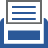printer friendly Reports: DNI950202-DNI9: Molecular Dynamics Simulation of Surfactant-Driven Wettability Alteration of Calcium Carbonate for Enhanced Oil Recovery

Seung Soon Jang, PhD, Georgia Institute of Technology

Narrative Progress Report

Background

Since ~ 50 % of the petroleum reserves are found in carbonate reservoirs and the average oil recovery from carbonate reservoirs through the water flooding method is often less than 30 %, the development of more efficient and productive technology for enhanced oil recovery (EOR) is very essential to fully utilize our energy resources as well as to reduce the energy consumption in producing a unit volume of petroleum from the reservoirs. In order to enhance the oil recovery from carbonate reservoirs, it is desirable to alter the wettability of the carbonate surface using surfactants from the oil-wet surface to the water-wet surface. However, the detailed mechanism of the wettability alteration has not been understood completely at molecular level, which should be a critical requisite in developing better EOR technology. Thus, we are investigating the molecular mechanisms of the surfactant-mediated wettability alteration on the carbonate surface.

Objectives

1. To predict probable structures of the carboxylate-adsorbed calcium carbonate surface using first principle density functional theory (DFT) calculations (1st year)

2. To develop a force field consisting of potential energy functions and parameters in order to simulate the surfactant-driven desorption of carboxylate from the calcium carbonate surface using molecular dynamics (MD) simulation (2nd year)

Computational Methods

All the spin-unrestricted DFT calculations in this study were performed using GGA in DMol3 with PBE functional and DNP numerical basis set. We used all-electron Kohn-Sham equation and. To calculate the charge number for atoms in molecule, Mulliken population was used in the Jaguar using B3LYP and 6-31G** basis set. The DRIEDING force filed was used to perform the energy minimization and equilibration of multi components system with new parameters to describe the interaction between carboxylate molecule and calcite surface with periodic boundary conditions in Cerius2.

Results and Discussions

1.  Decyltrimethylammonium Bromide (C10TAB)

This research included three types of molecule with various hydrophilicity. Hydrophilic molecules, such as water or benzoate, were created as full-atomistic model. On the other hand, the united-atom model was used to describe hydrophobic molecules, such as octane used to mimic oil phase. Based on these models, the hybrid model was employed to simulate amphiphilic molecules (C10TAB). As shown in Figure 1, the polar and hydrophilic head part of C10TAB uses full-atomistic model, whereas the hydrophobic tail part uses united-atom model.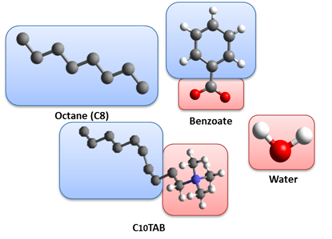Figure 1. Molecular structures of octane, benzoate, water, and C10TAB. The color of boxes in the background represents the hydrophilicity of specific parts (Blue: hydrophobic; Red: hydrophilic).

2.  Binding Energy Calculation

In this study, we investigate the adsorptive biding energy of benzoate on the calcite surface as defined in Equation (1), which is the difference on the energy between the total system and the component systems (Figure 2).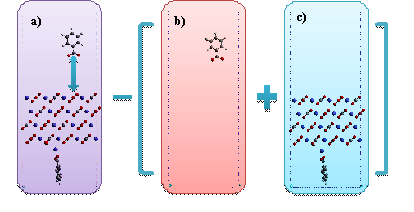Figure 2.  The demonstration of binding energy calculation. a) displays the system model used to calculate the total energy of system, which is electronically neutral, b) represents the molecule component model, the overall charge of which is negative one, and c) demonstrates the surface model, the overall charge of which is positive one. The cell parameters are 16.10•9.90•120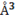.

In Figure 2, the overall electron charge of the total system zero because of +2 charge from a calcium atom and -2 charge from two benzoate molecules whereas the two component systems have non-zero charge states.  Therefore, we decided to consider the solvation effect to calculate the binding energy, which results in - 29.45 kcal/mol.

3.  Force Field Development

In this study, we developed new force fields to calculate the energy of the systems for MD simulation. The first task is to assign the force field (FF) type for all atoms used in the system to calculate van der Waals interactions. The FF type for calcite surface was already determined in the framework of MS-Q force field using the exponential 6 (exp-6) function (Equation (2)).

The FF for the interaction between benzoate molecule and calcite surface, however, has not been particularly developed. Considering the nature of the strong binding  of carboxylate group with the calcite surface (¥ÄEbinding = - 29.45 kcal/mol), we decided to develop a new FF using Morse potential function (Equation (3))

where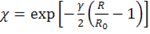for the carboxylate group in Figure 3.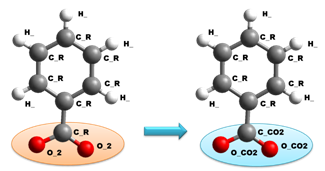Figure 3.  The new force field types for carboxylate to describe the adsorptive interaction with the calcite surface.

By performing multivariable optimization, we determined the FF parameters (Table 1) to reproduce the DFT binding energy curve as shown in Figure 4.

Table 1 List of FF parameters determined to reproduce the DFT adsorptive binding energy of benzoate molecule on the calcite surface

 Exp-6 Calcite (CaCO2) Aromatic Ring R0 D0 Ca_RC C_R 4.0778 0.2983 11.8213 C_RC 3.8972 0.09511 14.0337 O_RC 3.6368 0.09637 13.7477 Ca_RC H_ 3.5032 0.1524 10.6381 C_RC 3.5382 0.03760 13.2283 O_RC 3.3043 0.03783 12.9458 Morse Potential Calcite (CaCO2) Carboxylate R0 D0 g Ca_RC O_CO2 2.8639 0.267 13.099 C_RC 3.6003 0.0882 36.659 O_RC 7.8226 0.00100 6.521 Ca_RC C_CO2 4.2484 0.0100 0.784 C_RC 3.0553 0.0873 0.501 O_RC 3.5095 0.0600 0.602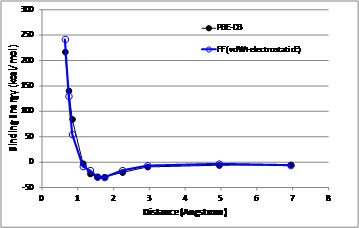Figure 4. The comparison of energy curves between the DFT modeling (black) and the FF modeling (blue).

Perspectives

Through this study, we investigated the adsorptive binding of benzoate (a model compound of carboxylate) using density functional theory with solvation effect, resulting in the binding energy of - 29.45 kcal/mol. Based on this DFT calculation, we developed a force field based on the Morse potential function which can reproduce the DFT energy curve. Using the new FF, we will simulate large-scale molecular layer on calcite surface with other molecular components such as surfactant, oil and water. In such large-scale MD simulations, we will evaluate the thermodynamic stability of adsorption of carboxylate molecule in the presence of oil and water by analyzing the structures and the energies. Furthermore, we will investigate the effect of the surfactant with various molecular structures on the carboxylate layer by evaluating the thermodynamic stability of molecular aggregation in comparison with that of the adsorbed carboxylate layer on calcite using MD simulation.

Word Count: 992 words

ND
Solid Flow
Instabilities
Dr. Hrenya
UNI
Ionic Liquid
Cage Effects